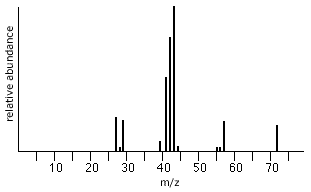# Why is the m/z value of the molecular ion important?

Dec 13, 2014

The m/z value of the molecular ion is important because it gives you the molecular mass of the compound.

You can use this information together with the fragmentation to figure out the structure of the compound.

For example, in the mass spectrum below, the m/z value of the molecular ion is 72.So the molecular mass is 72 u. The formula could be C₅H₁₂, C₄H₈O, C₃H₄O₂, or C₃H₈N₂.

We can assign some fragments as

72 = M
57 = M – 15 = CH₃
43 = M – 29 = CH₃CH₂
29 = CH₃CH₂ = M – CH₃CH₂CH₂

It looks as if the compound might be C₅H₁₂.

If you have a high-solution spectrometer, you can get the formula right away.

The isotopic masses are $\text{^1"H}$ = 1.0078 u; $\text{^12"C }$ = 12.0000 u; $\text{^14"N}$ = 14.0031 u;
$\text{^16"O}$ = 15.9949.u. Then the molecular masses are

C₅H₁₂ = 72.0936 u
C₄H₈O = 72.0573 u
C₃H₄O₂ = 72.0210 u
C₃H₈N₂ = 72.0686 u

If m/z = 72.0936, the formula of your compound is C₅H₁₂. You do not have to consider the other possible formulas.